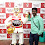### Common Errors in Secondary Mathematics

Common Errors Committed  by the  Students  in Secondary Mathematics   Errors  that students often make in doing secondary mathematics  during their practice and during the examinations  and their remedial measures are well explained here stp by step.  Some Common Errors in Mathematics

### Lesson Plan Math Class X (Ch-2) | Polynomials

E- LESSON PLAN   SUBJECT MATHEMATICS    CLASS 10
lesson plan for maths class 10 cbse, lesson plans for mathematics teachers,  Method to write lesson plan for maths class 10, lesson plan for maths class 10 real numbers, lesson plan for mathematics grade 10, lesson plan for maths in B.Ed.

 TEACHER  :   DINESH KUMAR SCHOOL :  RMB DAV CENTENARY PUBLIC SCHOOL NAWANSHAHR SUBJECT   :   MATHEMATICS CLASS                  :   X  STANDARDBOARD                 :  CBSE LESSON TOPIC / TITLE   : CHAPTER 1: POLYNOMIALS ESTIMATED DURATION: This lesson is divided into seven modules and it is completed in seven class meetings.

PRE- REQUISITE KNOWLEDGE:-

TEACHING AIDS:-

Green Board, Chalk,  Duster, Charts, smart board, projector and laptop  etc.
METHODOLOGY:-

Demonstration and Lecture method

LEARNING OBJECTIVES:-
• Basic concepts and definitions related to the topic polynomials.
• Explanation of remainder and factor theorem.
• Relationships between zeroes and coefficients of quadratic polynomials and cubic polynomials.
• Explanation of division algorithm.
• Method of finding all zeroes of cubic and bi-quadratic polynomials.

LEARNING OUTCOMES:
• After studying this lesson students will be able  to explain the relationship between zeroes and coefficients.
• Students should know the method of division of one polynomial with another and the division algorithm.
• Students should be able to factorize the quadratic, cubic and bi-quadratic polynomials and become able to find their zeroes.
RESOURCES
NCERT Text book of Mathematics
NCERT Exemplar Text Book
RESOURCE CENTRE at cbsemathematics.com

KEY WORDS :
Algebraic Expression, Polynomials, Degree of polynomials, Monomial, Binomial, Trinomial, Linear, Quadratic, Cubic, Bi-quadratic, Parabola, etc.

PROCEDURE & EXPLANATION

Start the session by checking their previous knowledge, by asking the questions related to the topic. After this explain the topic to the students.

Introduction and motivation

Teacher will start the session by giving many examples of Algebraic Expressions. Now teacher will explain which types of Algebraic Expressions are called Polynomials. After this teacher will introduce the definition of Polynomial and explain the different components of polynomials.

Polynomial: An algebraic expression in which the exponent of the variable is a whole number is called a polynomial.

Example: 3x+ 5,  4x- 8x+ 5x - 3,  5x+ 3x  are some examples of polynomial .

Important Definitions

Now teacher will explain the Important Definitions like Degree, coefficients and zeroes of the polynomials and their graphical representations.

Degree of constant polynomial is zero and degree of zero polynomial is undefined.

After this teacher will explain the different types of polynomial on the bases of degree and on the basis of number of terms.

Teacher will also give examples  and explanations of definitions of the polynomials like linear, quadratic, cubic, bi-quadratic, monomial, binomial, and tri-nomial .

Finding Zeroes from the Graph

Students will be given the knowledge of finding the number of zeroes from the graph of the polynomial.

Number of zeroes of a polynomial is equal to the number of times the graph of that polynomial meet the x - axis.

Graph of a quadratic polynomial is always parabolic.

Now teacher will explain the Factor method of finding the zeroes of quadratic polynomials and will explain the Relationship between zeroes and coefficients.

Teacher will also provide some problems to the students to check whether students can apply the method or not.

Now teacher will explain the method of finding the quadratic polynomial from the zeroes and explain the topic by taking some examples.

Division Algorithm

By taking some examples, teacher will explain the method of dividing one polynomial by another. To verify the result of division teacher will explain the Division Algorithm.

Dividend = Divisor  Quotient + Remainder

Now teacher will explain the method of finding all zeroes of bi quadratic polynomials by using factor theorem and division algorithm. Method will be well explained by taking examples.

Teacher can also give some problems to the students to check whether they will understand the concept or not.

Zeroes of Cubic Polynomial

Now concept of cubic polynomial is also be introduced. Students will be given the knowledge of cubic polynomial, its general form, its zeroes and the relationship between zeroes and coefficient.

STUDENTS DELIVERABLES:-
• Review questions given by the teacher.
• Student can prepare the presentation on the topic relationship between zeroes and coefficients for quadratic as well as cubic polynomials.
• Solve NCERT problems with examples,
• Solve assignment on Multiple Choice Questions (MCQ) given by the teacher.

MIND MAP
EXTENDED LEARNING:-

Students can extend their learning through the RESOURCE CENTRE and can find more valuable and interesting concepts on mathematics at  cbsemathematics.com

ASSESSMENT TECHNIQUES:-

Assignment sheet will be given as home work at the end of the topic. Separate sheets which will include questions of logical thinking and Higher order thinking skills will be given to the above average students.

Class Test , Oral Test , MCQ worksheet and Assignments can be made the part of assessment.
Re-test(s) will be conducted on the basis of the performance of the students in the test.

🙏

1.RAGHAVENDRA NAIK
Well planned lesson plan